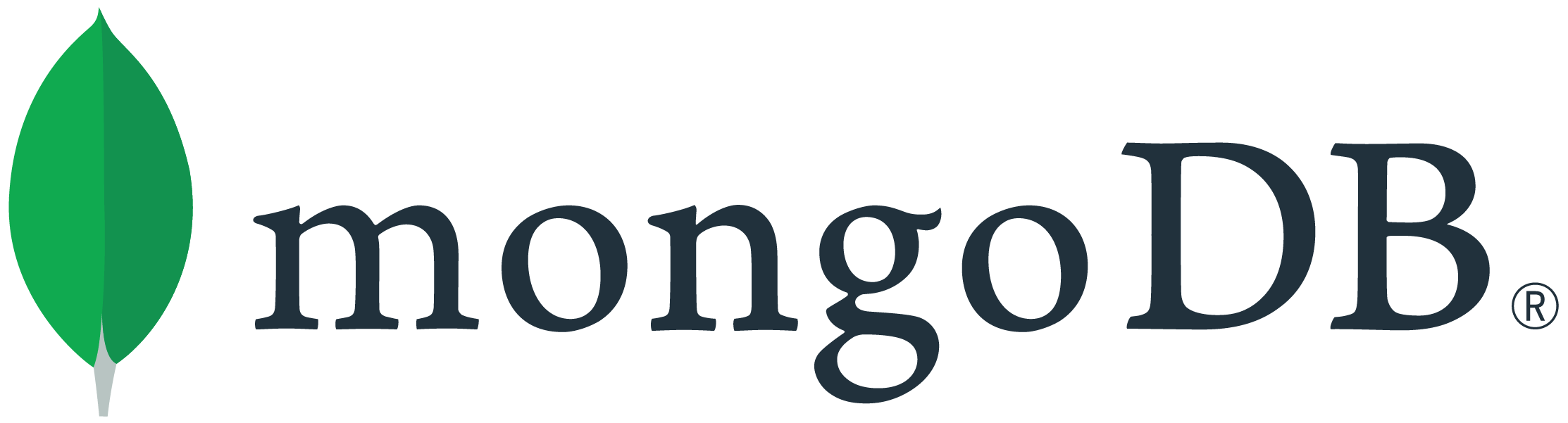# Possible Typo in Chapter 3 IDE directions

In the Chapter 3 IDE examples the value for class_id is 399. In the Chapter 3: Creating and Manipulating Documents Updating Documents - mongo shell the value is 339
There are no records with the class_id 399 and the given student_id’s

1. Find all documents in the grades collection where the student_id is 151,
and the class_id field is 399.
2. Find all documents in the grades collection where the student_id is 250,
and the class_id field is 399.
3. Update one document in the grades collection where the student_id is 250,
and the class_id field is 399, by adding a document element to the “scores”
array.

Thanks for surfacing this. We will update the questions in IDE lab.

~ Shubham

1 Like